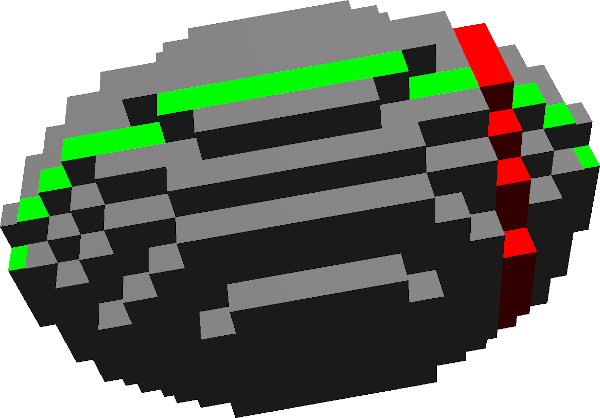DGtal  1.4.beta
Maximal segments and slices of digital surface based normal estimator

Author(s) of this documentation:
Jocelyn Meyron, Tristan Roussillon
Since
1.2

Part of the Geometry package.

This part of the manual describes the normal estimation algorithm based on the computation of maximal segment on slices of a digital surface (see  and ).

The following programs are related to this documentation: geometry/surfaces/exampleMaximalSegmentSliceEstimation.cpp, testArithmeticalDSSComputerOnSurfels.cpp.

# Introduction

Most of the estimators need some kind of parameters to control the neighborhood in which some averaging process is done. On the contrary, this estimator is interesting since it has no parameter. It is also fast and can be used as an input to other estimators (see PlaneProbingDigitalSurfaceLocalEstimator for instance). The idea is the following (for a surfel $$s$$):

1. We compute the two 2-dimensional slices of the digital surface for the two directions of the surfel (using the DigitalSurface2DSlice class).
2. For each slice, we project every surfel on a plane that is orthogonal to the initial surfel. This gives us two sets of 2D points.
3. On each one of these sets, we use a recognition algorithm like the ones presented in Recognition of digital straight segments to compute the leftmost and rightmost maximal segments. This gives us two 2D normal vectors that we average.
4. We finally take the cross product of the two 2D normal vectors.Two slices on the digitization of an ellipsoid

# Usage

The estimation is implemented in the class MaximalSegmentSliceEstimation. It is a model of concepts::CSurfelLocalEstimator and concepts::CDigitalSurfaceLocalEstimator, so it has the following methods:

It can be instantiated and used as follows:

// Instantiation
using Estimator = MaximalSegmentSliceEstimation<Surface>;
Estimator estimator;
estimator.init(gridstep, surfels.begin(), surfels.end());
estimator.attach(surface);
// Usage
std::vector<Estimator::Quantity> quantities;
estimator.eval(surfels.begin(), surfels.end(), std::back_inserter(quantities));
PlaneProbingParallelepipedEstimator< DigitalPlane, ProbingMode::R1 > Estimator

# Implementation details

The class is essentially a wrapper of the class ArithmeticalDSSComputerOnSurfels which is an adaptation of ArithmeticalDSSComputer to recognize 2D digital segments on projections of 3D surfels. It can be instantiated as follows:

// Defining the type
using Container = std::vector<Surfel>;
using Integer = int;
using SegmentComputerOnSurfels = ArithmeticalDSSComputerOnSurfels<KSpace, Container::const_iterator, Integer, adjacency>;
// Instantiation
SegmentComputerOnSurfels computer(kspace, // A 3D Khalimsky space
dim1, // The first dimension to project onto
dim2 // The second dimension to project onto
);
Aim: This class is a wrapper around ArithmeticalDSS that is devoted to the dynamic recognition of dig...
Z3i::SCell SCell
Point::Coordinate Integer
KhalimskySpaceND< 3, Integer > KSpace
Definition: StdDefs.h:146

See the page Digital straight lines and segments
for more details on how to use it.